Home PHYSICS TOPIC 5: SIMPLE MACHINES | PHYSICS FORM 1

TOPIC 5: SIMPLE MACHINES | PHYSICS FORM 1

152
0
SHARESimple Machines

A simple machine is a non-powered mechanical device that changes the direction or magnitude of a force. In general, they can be defined as the simplest mechanisms that use mechanical advantage (also called leverage) to multiply force. A simple machine uses a single applied force to do work against a single load force. Ignoring friction losses, the work done on the load is equal to the work done by the applied force.
Nowadays man can do many things without necessarily using much of his own energy. Many can fly by using aeroplane, raise a heavy load, and drive a nail into wood by using a hammer. All of these are achieved using machines.
The Concept of a Simple Machine
Explain the concept of a simple machine

Machine:

Machine is any device, which is used for simplifying work. Examples of machines are: a crowbar, a seesaw, a claw hammer, a pulley and an inclined plane.
Types of machine:
There are two types of machines
1. Simple machine
2. Complex machine
What is simple machine?
Simple
machine is any device, which requires single force in operation to
simplify work e.g. Claw hammer, a pulley, and an inclined plane. In a
simple machine, a force is applied at one convenient point to overcome
another force acting at another point.
Simple MachineThe
diagram above shows a stone being shifted. Force is applied at one end
of the bar in order to exert an upward force on the stone. The down ward
force is called effort and the weight of the stone is called load.
Effort is the force used to operate a machine. And Load is the resistance, which machines overcome.
The terms Applied in Simple Machine
Explain the terms applied in simple machine
Terms used in simple machine
Mathematically:Mechanical advantage has no SI unit.
Example 1
Example 1
A simple machine raises a load of 100N by using a force 50N. Calculate the mechanical advantage.
Solution;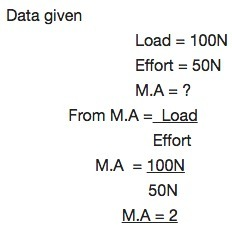Example 2
Example 2.
A force of 20N raises a load of 100kg. Calculate mechanical advantage of the machine.
Solution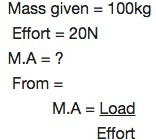img4Velocity ratio
Velocity
ratio is the ratio of distance travelled by effort and distance
travelled by load. Or Velocity ratio is the distance moved by effort per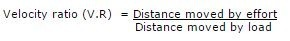Example 3
Example
In
a certain machine a force of 10N moves down a distance of 5cm in order
to raise a load of 100N through a height of 0.5cm calculated the
velocity ratio (V.R) of the machine.
Solution:
Distance by Effort = 5cm
Distance moved by load = 0.5cm.
From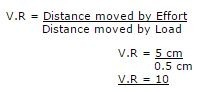Efficiency of Machine
Efficiency of machine is the ratio of work output and work input.
Work input = Effort x Distance moved by Effort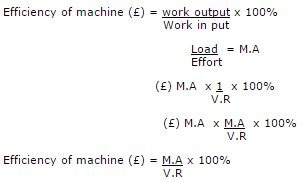A perfect machine has 100% Efficiency. Therefore M.A is equal to V.R.
Note:
most machines are imperfect machines since efficiency is less than 100%
this is due to the friction and heat and loss of energy.
Example 4
Example1:
A certain machine with force of 10N moves down a distance of 5cm in order to raise a load of 100N through a height of 0.5cm.
Calculate:
1. M.A
2. V.R
3. Efficiency of machine
Solution:
Date given:Effort = 10N
Distance moved by effort = 5cm
Efficiency machine?
Efficiency of machine () = M.A x 100%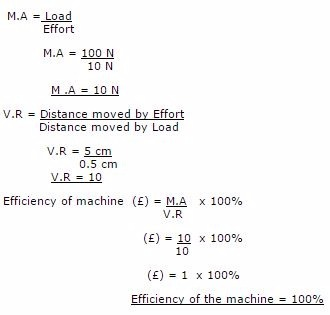Different Kinds of Simple Machine
Identify the different kinds of simple machines
Different Kinds of Simple Machines
Types of Simple Machines
• LEVERS
• PULLEYS
• INCLINED PLANE
• THE SCREW AND SCREW JACK
• WHEEL AND AXLE
• HYDRAULIC PRESS
SHARE
Previous articleTOPIC 4: FORCES IN EQUILIBRIUM | PHYSICS FORM 1